# SQL 中 Left Join 转为 Inner Join 的实际应用

#### 需求说明：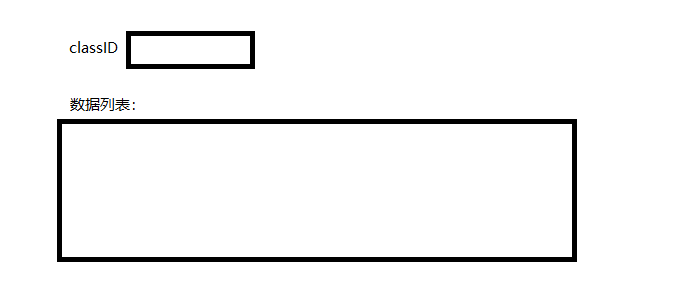SELECT *
FROM T_CLASS A
LEFT JOIN T_STUDENT B
ON A.CLASS_ID = B.CLASS_ID
AND B.SEX = 1
ORDER BY A.CLASS_ID;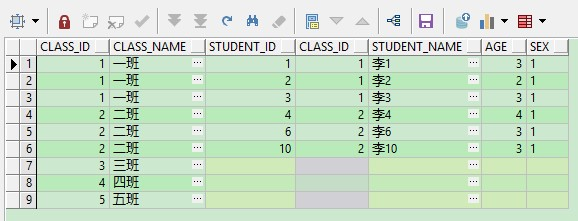SELECT *
FROM T_CLASS A
LEFT JOIN T_STUDENT B
ON A.CLASS_ID = B.CLASS_ID
AND B.SEX = 1
AND A.CLASS_ID = 1
ORDER BY A.CLASS_ID;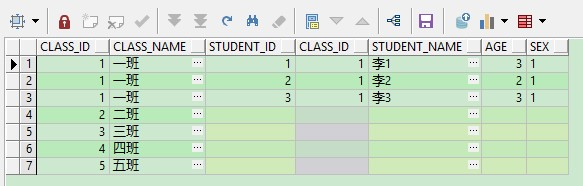##### 第一种，直接将左关联改为内关联，即将 Left Join 改为 Inner Join
SELECT *
FROM T_CLASS A
INNER JOIN T_STUDENT B
ON A.CLASS_ID = B.CLASS_ID
AND B.SEX = 1
AND A.CLASS_ID = 1
ORDER BY A.CLASS_ID;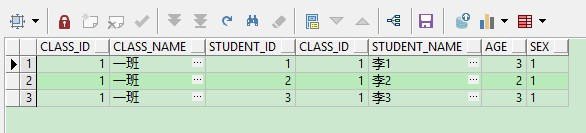##### 第二种，用 where 进行过滤，查询语句如下：
SELECT *
FROM T_CLASS A
INNER JOIN T_STUDENT B
ON A.CLASS_ID = B.CLASS_ID
AND B.SEX = 1
WHERE A.CLASS_ID = 1
ORDER BY A.CLASS_ID;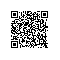Java技术进阶使用钉钉扫一扫加入圈子
+ 订阅

Java技术进阶成长，课程资料，案例解析，实战经验全都有！